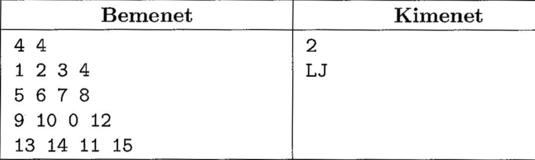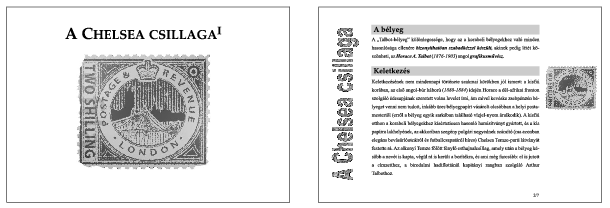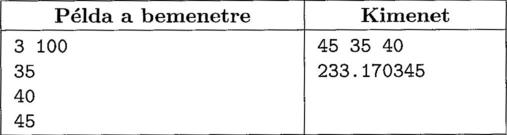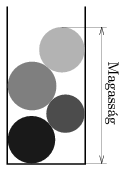Mathematical and Physical Journal
for High Schools
Issued by the MATFUND Foundation
 Already signed up? New to KöMaL?

# KöMaL Problems in Informatics, April 2011

Show/hide problems of signs:## Problems with sign 'I'

Deadline expired on May 10, 2011.

I. 265. Many of us know the 15 puzzle, where 15 tiles are shuffled and there is 1 empty square on a $\displaystyle 4\times 4$ board. During the game one can move any neighboring tile into the empty square. The goal is to put all the 15 tiles into a predefined order, that is, into an ascending order from left to right and from top to bottom.

The original $\displaystyle 4\times 4$ version can be played on an $\displaystyle N\times M$ board with the same rules.

Your program should give the steps recovering the original state of the $\displaystyle N\times M$ board. The first command line argument to your program is the name of the input file, while the second argument is the name of the output file.

The first line of the input file contains the values $\displaystyle N\le 20$ and $\displaystyle M\le 20$ separated by a space. Then each of the following $\displaystyle N$ lines contain $\displaystyle M$ numbers, separated by a space, describing a shuffled puzzle. The empty square is denoted by a 0.

The first line of the output file contains the (not necessary minimal) number of steps needed to recover the board from the shuffled state. The second line of the output file should contain exactly this number of characters, consisting of letters B, F, J or L. These letters describe in each step the direction from which we move the tile to the empty square (B -- left, F -- above, J -- right, L -- below). If the correct final order can not be realized, the first line of the output should be $\displaystyle -1$.

In the example, Bemenet is the input, while Kimenet is a possible output.The source code (i265.pas, i265.cpp, ...) together with a short documentation (i265.txt, i265.pdf, ...) -- also describing which developer environment to use for compiling, further a brief description of your solution -- should be submitted.

(10 pont)

solution (in Hungarian), statistics

I. 266. Many computer programs use the special case of the linear congruence method to generate random numbers''.

Suppose we are given k integers (a1,a2,...,ak), being the coefficients of the congruence. We are also give k initial values (R1,R2,...,Rk). The next pseudo random number is then defined recursively according to the formula. It is a natural expectation that the generated pseudo random integers sooner or later attain every possible value and we should not be able to guess the next term of the sequence based on the previous terms.

By using a spreadsheet application and the linear congruence method above, create 1000 pseudo random numbers and compare them with the random numbers generated by the spreadsheet application. Use the value k=10 (being the length of the coefficient vector, and the initial value vector). Use M=50 (hence the pseudo random integers are distributed in the interval [0,49]). Use the columns A:K to generate the random numbers. The coefficients and initial values should be put in the first row. These numbers are arbitrary two-digit integers specified by us.

In order to do the comparison, first use the random numbers in column K with references and make pairs in the column N:O. Then, by interpreting these as coordinate pairs, graph them on a PointXY diagram. This diagram should not contain any legend and the points should fill in the available space. The other column P:Q for the comparison should consist of similar pairs of random numbers obtained by the built-in random number generator of the spreadsheet application. These numbers should be graphed on a similar but different diagram.

As a second method for the comparison, you should count how many times each number occurs. This should be done to the right of column Q: first by using your random numbers, then in a different column, the random numbers of the spreadsheet application. You should sort these occurrences into ascending order and plot them together on a column chart. Correct results should appear even if the sheet is recomputed.

Your spreadsheet (i266.xls, i266.ods, ...) should be submitted in a compressed file (i266.zip) together with a short documentation (i266.txt, i266.pdf, ...) also containing the name and version number of your spreadsheet application and a brief description of your solution.

(10 pont)

solution (in Hungarian), statistics

I. 267. In this task you should create a document having the same content and format as the file chelsea_minta.pdf downloadable from our website. All the necessary files are found in the compressed file chelsea_forras.zip.

The document uses A4 paper format with 6 cm left margin, and 7 cm right margin. You should notice that some characters can not be found in the source file.

Your solution gets more points if it makes use of the features of the word processor providing effective and seamless workflow.

Your document should be submitted in the default format of the word processor (i267.doc, i267.docx, i267.ods, ...) together with a description (i267.txt, i267.pdf, ...) containing the name and version of the word processor.(10 pont)

solution (in Hungarian), statistics## Problems with sign 'S'

Deadline expired on May 10, 2011.

S. 62. Some balls are dropped into a cylinder-shaped box. Your program s62 should determine the order in which their total height is minimal. (In the example, Magasság'' is height.)

The number of balls, the diameter of the cylinder and the radii of the balls are read from the input file. The result -- that is, the order of the balls and the minimal height -- is displayed on the screen to 6 digits precision. The name of the input file is a command line argument. In the example, Példa a bemenetre'' is a sample input, while Kimenet'' is the output.

The first line of the input contains the number of balls ($\displaystyle 3\le n\le 30)$ and the diameter of the cylinder ($\displaystyle 10\le d\le 100)$. The following $\displaystyle n$ lines contain the radius of the balls ($\displaystyle d/3<r<d/2)$.The source code and project files of your solution -- without the .exe or any other auxiliary files generated by the compiler -- should be submitted together with a brief description of your solution (s62.txt, s62.pdf, ...) in a compressed file s62.zip. (This exercise is based on the exercise Project Euler #222.)(10 pont)

solution (in Hungarian), statistics

\$Var(Body)